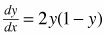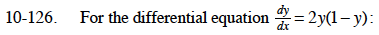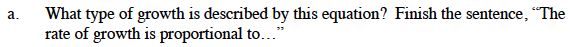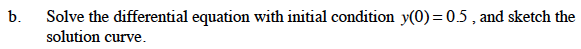### Home > CALC > Chapter 10 > Lesson 10.3.2 > Problem10-126

10-126.
1. For the differential equation: Homework Help ✎

1. What type of growth is described by this equation? Finish the sentence, "The rate of growth is proportional to…"

2. Solve the differential equation with initial condition y(0) = 0.5, and sketch the solution curve.... the product of ___ and ___.By partial fraction decomposition:

Solve for the value of A: